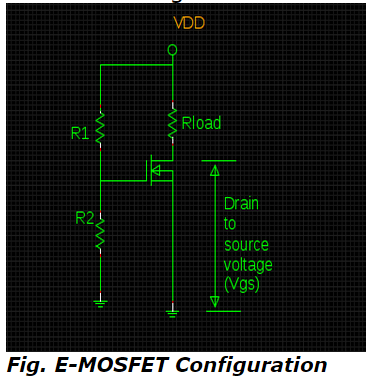# Static Characteristics of MOSFET - MCQs with answers

## Static Characteristics of MOSFET - MCQs with answers

Q1. The input current of JFET and MOSFET are basically the leakage currents of ______ & ______ respectively

a. forward-biased PN junction & capacitor
b. reversed-biased PN junction & capacitor
c. forward-biased PN junction & inductor
d. reversed-biased PN junction & inductor

ANSWER: b. reversed-biased PN junction & capacitor

Q2. How is the layer of P-substrate, that intimate the provision of channel for electrons by touching substrate to metal -oxide film with the sementic behaviour of N-channel, can be referred as ?

a. N-type Inversion layer
b. P-type Inversion layer
c. Both a & b
d. None

Q3. What is the computational the value of drain current for gate-to-source voltage (VGS ) of about 6V in the below drawn schematic diagram of E-MOSFET along with ID(ON) = 2mA at VGS & VGS(threshold) of about 12V & 4V respectively?a. 1 mA
b. 2 mA
c. 3 mA
d.4 mA